• ## 手写数字识别

千人学习 2017-06-28 20:34:23
手写数字识别，使用简化后的近邻算法实现手写数字识别c++小游戏 c++游戏 c++链表
• ## 手写字体识别

千次阅读 2019-07-10 13:40:28
1.完成手写字体识别kaggle比赛：https://www.kaggle.com/c/digit-recognizer 2.环境：谷歌云盘（内有GPU运算，速度比我自己电脑快好多倍） 3.手写字体文件 train.csv文件第一列是图像标签，后面784(28*28)列是...
1.完成手写字体识别kaggle比赛：https://www.kaggle.com/c/digit-recognizer

2.环境：谷歌云盘（内有GPU运算，速度比我自己电脑快好多倍）

3.手写字体文件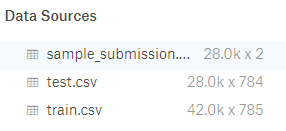train.csv文件第一列是图像标签，后面784(28*28)列是像素。

test.csv文件是784列像素。

sample_submission.csv是提交的文件

4.谷歌云盘启动

from google.colab import drive
drive.mount('/content/drive/')
!ls "/content/drive/My Drive/"
import tensorflow as tf
tf.test.gpu_device_name()
!ls
# 切换目录
import os
os.chdir("/content/drive/My Drive/home")
!ls

5.预处理图像

# 预处理数据
import keras
import pandas as pd
import numpy as np
from keras.utils import np_utils
dataset = np.array(dataset)
x = dataset[:,1:]
x = x/255
x = x.reshape(-1,28,28,1)
y = dataset[:,0]
y = np_utils.to_categorical(y)
print(x.shape)
print(y.shape)

6.构建模型训练

from keras.layers.convolutional import Conv2D, MaxPooling2D
from keras.layers import *
from keras.models import Sequential
def creat_model():
model = Sequential()
model.compile(loss='categorical_crossentropy', optimizer='sgd', metrics=['accuracy'])
return model
model = creat_model()
print(model.summary())
model.fit(x=x,y=y,epochs=10,batch_size=20)

7.数据预测

dataset2 = pd.read_csv('test.csv')
dataset2 = np.array(dataset2)
dataset2 = dataset2.reshape(-1,28,28,1)
print(dataset2.shape)
y = model.predict(dataset2)
print(y.shape)
y = np.argmax(y,axis=1)
test = np.array(test)
for i in range(len(y)):
test[i] = y[i]
test = pd.DataFrame(test,columns=['ImageId', 'Label'])
print(test)
test.to_csv('sample_submission.csv',index=False)

8.比赛成绩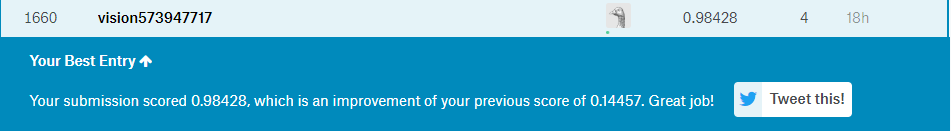准确率98.428%，那个模型训练多一下次数准确率应该可以去到更好。

9.项目截图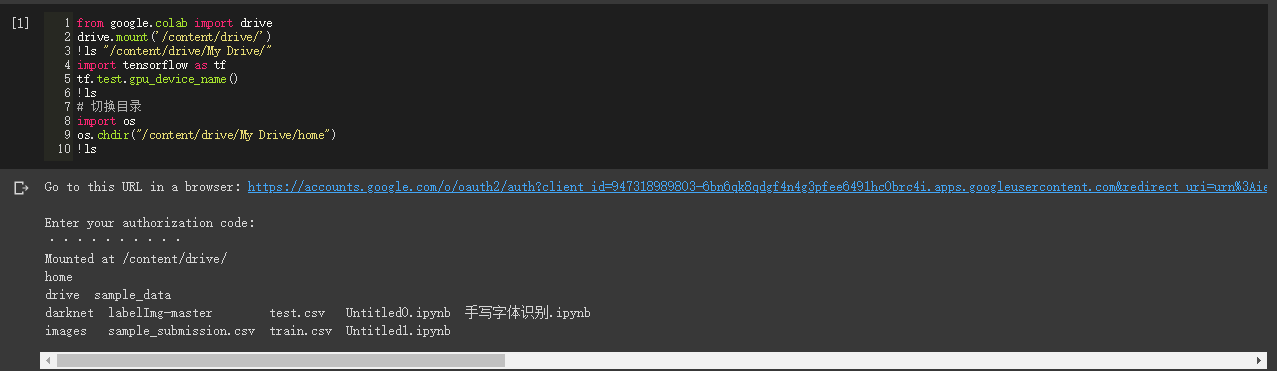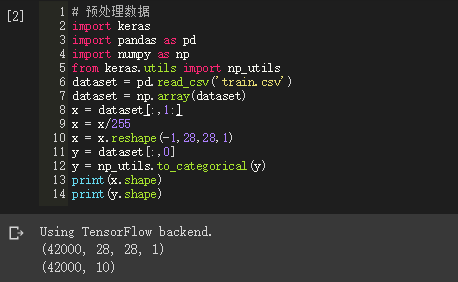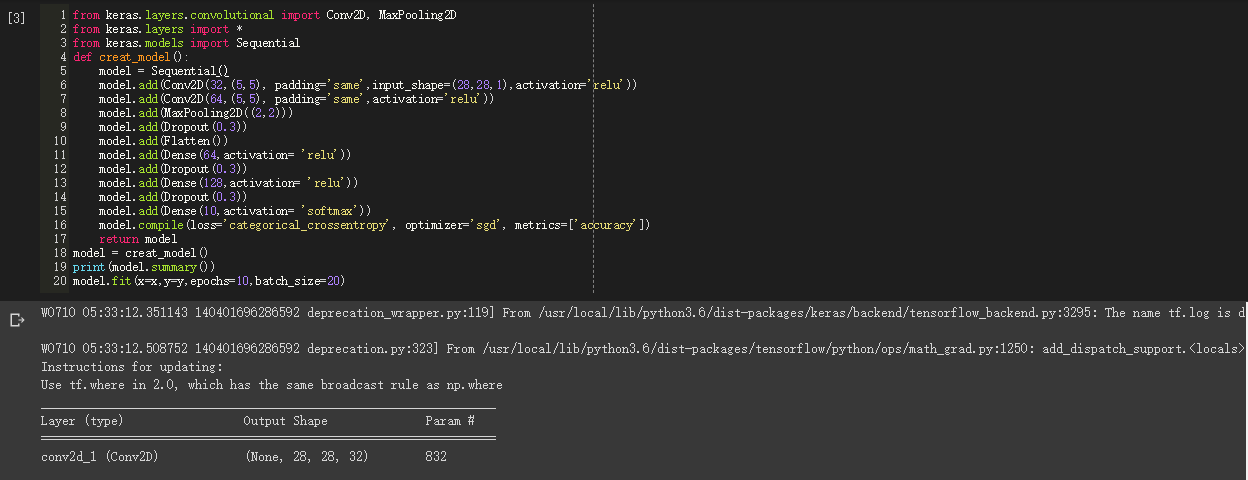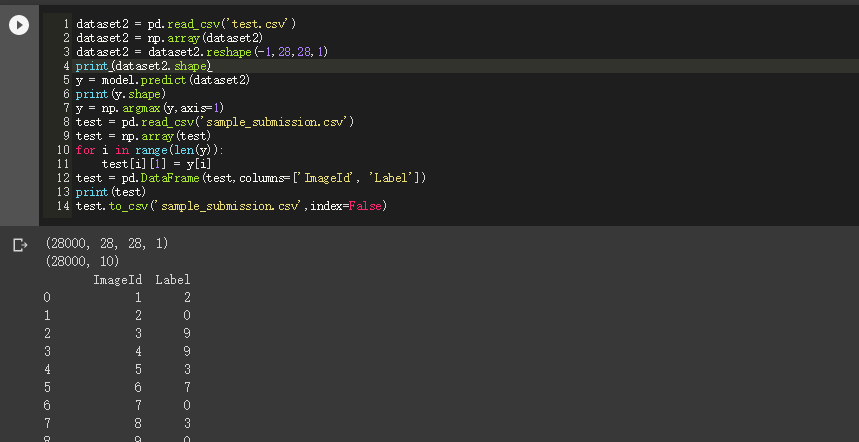展开全文• 本文使用Tensorflow框架进行Python编程实现基于卷积神经网络的手写数字识别算法，并将其封装在一个GUI界面中，最终，设计并实现了一个手写数字识别系统。
手写数字识别算法的设计与实现
本文使用python基于TensorFlow设计手写数字识别算法，并编程实现GUI界面，构建手写数字识别系统。这是本人的本科毕业论文课题，当然，这个也是机器学习的基本问题。本博文不会以论文的形式展现，而是以编程实战完成机器学习项目的角度去描述。

项目要求：本文主要解决的问题是手写数字识别，最终要完成一个识别系统。
设计识别率高的算法，实现快速识别的系统。
1 LeNet-5模型的介绍
本文实现手写数字识别，使用的是卷积神经网络，建模思想来自LeNet-5，如下图所示：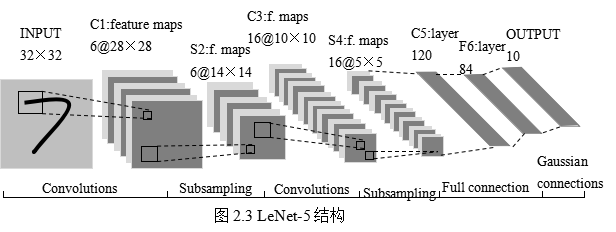这是原始的应用于手写数字识别的网络，我认为这也是最简单的深度网络。
LeNet-5不包括输入，一共7层，较低层由卷积层和最大池化层交替构成，更高层则是全连接和高斯连接。
LeNet-5的输入与BP神经网路的不一样。这里假设图像是黑白的，那么LeNet-5的输入是一个32*32的二维矩阵。同时，输入与下一层并不是全连接的，而是进行稀疏连接。本层每个神经元的输入来自于前一层神经元的局部区域(5×5)，卷积核对原始图像卷积的结果加上相应的阈值，得出的结果再经过激活函数处理，输出即形成卷积层（C层）。卷积层中的每个特征映射都各自共享权重和阈值，这样能大大减少训练开销。降采样层（S层）为减少数据量同时保存有用信息，进行亚抽样。
第一个卷积层（C1层）由6个特征映射构成，每个特征映射是一个28×28的神经元阵列，其中每个神经元负责从5×5的区域通过卷积滤波器提取局部特征。一般情况下，滤波器数量越多，就会得出越多的特征映射，反映越多的原始图像的特征。本层训练参数共6×(5×5+1)=156个，每个像素点都是由上层5×5=25个像素点和1个阈值连接计算所得，共28×28×156=122304个连接。
S2层是对应上述6个特征映射的降采样层（pooling层）。pooling层的实现方法有两种，分别是max-pooling和mean-pooling，LeNet-5采用的是mean-pooling，即取n×n区域内像素的均值。C1通过2×2的窗口区域像素求均值再加上本层的阈值，然后经过激活函数的处理，得到S2层。pooling的实现，在保存图片信息的基础上，减少了权重参数，降低了计算成本，还能控制过拟合。本层学习参数共有1*6+6=12个，S2中的每个像素都与C1层中的2×2个像素和1个阈值相连，共6×(2×2+1)×14×14=5880个连接。
S2层和C3层的连接比较复杂。C3卷积层是由16个大小为10×10的特征映射组成的，当中的每个特征映射与S2层的若干个特征映射的局部感受野（大小为5×5）相连。其中，前6个特征映射与S2层连续3个特征映射相连，后面接着的6个映射与S2层的连续的4个特征映射相连，然后的3个特征映射与S2层不连续的4个特征映射相连，最后一个映射与S2层的所有特征映射相连。此处卷积核大小为5×5，所以学习参数共有6×(3×5×5+1)+9×(4×5×5+1)+1×(6×5×5+1)=1516个参数。而图像大小为28×28，因此共有151600个连接。
S4层是对C3层进行的降采样，与S2同理，学习参数有16×1+16=32个，同时共有16×(2×2+1)×5×5=2000个连接。
C5层是由120个大小为1×1的特征映射组成的卷积层，而且S4层与C5层是全连接的，因此学习参数总个数为120×(16×25+1)=48120个。
F6是与C5全连接的84个神经元，所以共有84×(120+1)=10164个学习参数。
卷积神经网络通过通过稀疏连接和共享权重和阈值，大大减少了计算的开销，同时，pooling的实现，一定程度上减少了过拟合问题的出现，非常适合用于图像的处理和识别。
2 手写数字识别算法模型的构建
2.1 各层设计
有了第一节的基础知识，在这基础上，进行完善和改进。

输入层设计

输入为28×28的矩阵，而不是向量。

激活函数的选取

Sigmoid函数具有光滑性、鲁棒性和其导数可用自身表示的优点，但其运算涉及指数运算，反向传播求误差梯度时，求导又涉及乘除运算，计算量相对较大。同时，针对本文构建的含有两层卷积层和降采样层，由于sgmoid函数自身的特性，在反向传播时，很容易出现梯度消失的情况，从而难以完成网络的训练。因此，本文设计的网络使用ReLU函数作为激活函数。
ReLU的表达式：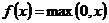卷积层设计

本文设计卷积神经网络采取的是离散卷积，卷积步长为1，即水平和垂直方向每次运算完，移动一个像素。卷积核大小为5×5。

降采样层

本文降采样层的pooling方式是max-pooling，大小为2×2。

输出层设计

输出层设置为10个神经网络节点。数字0~9的目标向量如下表所示：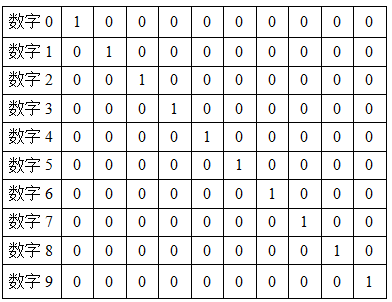2.2 网络模型的总体结构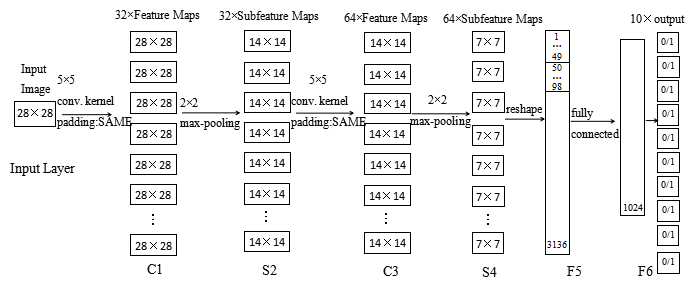其实，本文网络的构建，参考自TensorFlow的手写数字识别的官方教程的，读者有兴趣也可以详细阅读。
2.3 编程实现算法
本文使用Python，调用TensorFlow的api完成手写数字识别的算法。
注：本文程序运行环境是：Win10,python3.5.2。当然，也可以在Linux下运行，由于TensorFlow对py2和py3兼容得比较好，在Linux下可以在python2.7中运行。
#!/usr/bin/env python2
# -*- coding: utf-8 -*-
"""
Created on Fri Feb 17 19:50:49 2017

@author: Yonghao Huang
"""

#import modules
import numpy as np
import matplotlib.pyplot as plt
import tensorflow as tf
import time
from datetime import timedelta
import math
from tensorflow.examples.tutorials.mnist import input_data

def new_weights(shape):
return tf.Variable(tf.truncated_normal(shape,stddev=0.05))
def new_biases(length):
return tf.Variable(tf.constant(0.1,shape=length))
def conv2d(x,W):
def max_pool_2x2(inputx):

#import data
data = input_data.read_data_sets("./data", one_hot=True)  # one_hot means [0 0 1 0 0 0 0 0 0 0] stands for 2

print("Size of:")
print("--Training-set:\t\t{}".format(len(data.train.labels)))
print("--Testing-set:\t\t{}".format(len(data.test.labels)))
print("--Validation-set:\t\t{}".format(len(data.validation.labels)))
data.test.cls = np.argmax(data.test.labels,axis=1)   # show the real test labels:  [7 2 1 ..., 4 5 6], 10000values

x = tf.placeholder("float",shape=[None,784],name='x')
x_image = tf.reshape(x,[-1,28,28,1])

y_true = tf.placeholder("float",shape=[None,10],name='y_true')
y_true_cls = tf.argmax(y_true,dimension=1)
# Conv 1
layer_conv1 = {"weights":new_weights([5,5,1,32]),
"biases":new_biases()}
h_conv1 = tf.nn.relu(conv2d(x_image,layer_conv1["weights"])+layer_conv1["biases"])
h_pool1 = max_pool_2x2(h_conv1)
# Conv 2
layer_conv2 = {"weights":new_weights([5,5,32,64]),
"biases":new_biases()}
h_conv2 = tf.nn.relu(conv2d(h_pool1,layer_conv2["weights"])+layer_conv2["biases"])
h_pool2 = max_pool_2x2(h_conv2)
# Full-connected layer 1
fc1_layer = {"weights":new_weights([7*7*64,1024]),
"biases":new_biases()}
h_pool2_flat = tf.reshape(h_pool2,[-1,7*7*64])
h_fc1 = tf.nn.relu(tf.matmul(h_pool2_flat,fc1_layer["weights"])+fc1_layer["biases"])
# Droupout Layer
keep_prob = tf.placeholder("float")
h_fc1_drop = tf.nn.dropout(h_fc1,keep_prob)
# Full-connected layer 2
fc2_layer = {"weights":new_weights([1024,10]),
"biases":new_weights()}
# Predicted class
y_pred = tf.nn.softmax(tf.matmul(h_fc1_drop,fc2_layer["weights"])+fc2_layer["biases"])  # The output is like [0 0 1 0 0 0 0 0 0 0]
y_pred_cls = tf.argmax(y_pred,dimension=1)  # Show the real predict number like '2'
# cost function to be optimized
cross_entropy = -tf.reduce_mean(y_true*tf.log(y_pred))
# Performance Measures
correct_prediction = tf.equal(y_pred_cls,y_true_cls)
accuracy = tf.reduce_mean(tf.cast(correct_prediction,"float"))
with tf.Session() as sess:
init = tf.global_variables_initializer()
sess.run(init)
train_batch_size = 50
def optimize(num_iterations):
total_iterations=0
start_time = time.time()
for i in range(total_iterations,total_iterations+num_iterations):
x_batch,y_true_batch = data.train.next_batch(train_batch_size)
feed_dict_train_op = {x:x_batch,y_true:y_true_batch,keep_prob:0.5}
feed_dict_train = {x:x_batch,y_true:y_true_batch,keep_prob:1.0}
sess.run(optimizer,feed_dict=feed_dict_train_op)
# Print status every 100 iterations.
if i%100==0:
# Calculate the accuracy on the training-set.
acc = sess.run(accuracy,feed_dict=feed_dict_train)
# Message for printing.
msg = "Optimization Iteration:{0:>6}, Training Accuracy: {1:>6.1%}"
# Print it.
print(msg.format(i+1,acc))
# Update the total number of iterations performed
total_iterations += num_iterations
# Ending time
end_time = time.time()
# Difference between start and end_times.
time_dif = end_time-start_time
# Print the time-usage
print("Time usage:"+str(timedelta(seconds=int(round(time_dif)))))
test_batch_size = 256
def print_test_accuracy():
# Number of images in the test-set.
num_test = len(data.test.images)
cls_pred = np.zeros(shape=num_test,dtype=np.int)
i = 0
while i < num_test:
# The ending index for the next batch is denoted j.
j = min(i+test_batch_size,num_test)
# Get the images from the test-set between index i and j
images = data.test.images[i:j, :]
# Get the associated labels
labels = data.test.labels[i:j, :]
# Create a feed-dict with these images and labels.
feed_dict={x:images,y_true:labels,keep_prob:1.0}
# Calculate the predicted class using Tensorflow.
cls_pred[i:j] = sess.run(y_pred_cls,feed_dict=feed_dict)
# Set the start-index for the next batch to the
# end-index of the current batch
i = j
cls_true = data.test.cls
correct = (cls_true==cls_pred)
correct_sum = correct.sum()
acc = float(correct_sum) / num_test
# Print the accuracy
msg = "Accuracy on Test-Set: {0:.1%} ({1}/{2})"
print(msg.format(acc,correct_sum,num_test))
# Performance after 10000 optimization iterations


运行结果显示：测试集中准确率大概为99.2%。
我还写了一些辅助函数，可以查看部分识别错误的图片，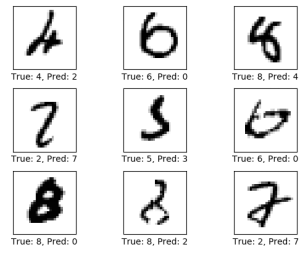还可以查看混淆矩阵，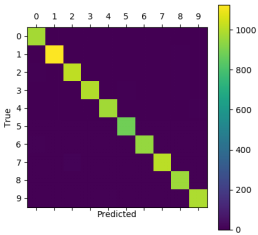2.3 实现手写识别系统
最后，将训练好的参数保存，封装进一个GUI界面中，形成一个手写识别系统。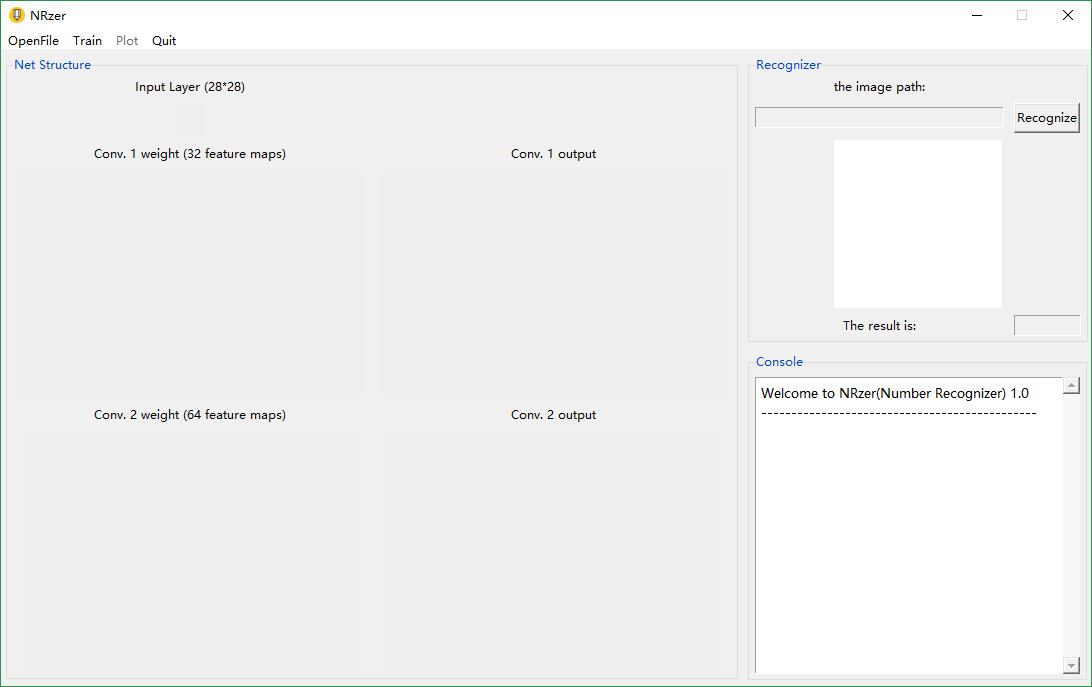系统中还添加了一点图像预处理的操作，比如灰度化，图像信息的归一化等，更贴近实际应用。
系统可进行快速识别，如下图：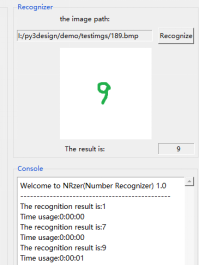3 总结
本文实现的系统其实是基于卷积神经网络的手写数字识别系统。该系统能快速实现手写数字识别，成功识别率高。缺点：只能正确识别单个数字，图像预处理还不够，没有进行图像分割，读者也可以自行添加，进行完善。
4 收获
本人之前的本科期间，虽然努力学习高数、线性代数和概率论，但是没有认真学习过机器学习，本人是2017年才开始系统学习机器学习相关知识，而且本科毕业论文也选择了相关的课题，虽然比较基础，但是认真完成后，有一种学以致用的满足感，同时也激励着我进行更深入的理论学习和实践探讨，与所有读者共勉。
==================================
2018年5月13日更新
以上是基本网络的设计与基本的实现，可满足入门学习。
相关链接:

========================================
2018年6月6日更新更新！！
python(TensorFlow)实现手写字符识别

此处的“手写字符”，其实指的是notMNIST数据库中的手写字符，其实和MNIST数据库是一样的。这里实现手写字符识别，主要是展示TensorFlow框架的可拓展性很强，具体来说，就是可以通过改动少部分的代码，从而实现一个新的识别功能。

NotMnist数据库
这个数据库和MNIST数据库基本一样，只是把10个数字换成了10个字母，即：A,B,C,D,E,F,G,H,I,J,K
当然，这个数据库的识别难度大一些，因为数据噪声更多一些，详情读者可以搜一搜了解一下。
实战
将NotMNIST数据库下载以后，放在本博文上述的网络中，基本不需要修改代码，直接训练，即可得到一个能识别字符的网络模型。
最后在测试集中的准确率，比MNIST的会低一些，大概为96%左右。
本文也将训练好的网络模型封装在和上述系统相似的GUI系统中，
[外链图片转存失败(img-k7xPyAio-1564543116627)(https://i.imgur.com/59M3NlD.png)]
识别效果还可以！
同样，将卷积卷积层可视化。
[外链图片转存失败(img-tIWWgZB9-1564543116629)(https://i.imgur.com/4awe7NY.png)]
结语
TensorFlow框架可拓展性很强，只要设计好了网络，就能很容易的实现出来；同时，使用基本的CNN识别整体架构也是大同小异的，很多识别任务是通用的。当然，在具体的实践中需要得到接近完美的效果，还是要下很大功夫的！努力学习吧，加油！
（如果你/您有什么有趣的想法，可以在下面留言，如果我也感兴趣同时又有时间的话，我会尝试做一做，_）


展开全文机器学习 python Tensorflow
• import os import torch import torch.nn as nn import torch.utils.data as Data import torchvision import matplotlib.pyplot as plt # torch.manual_seed(1) # reproducible # Hyper Parameters ...python
• matlab 图像处理，基于matlab的图像处理手写字识别系统
• 你知道手写文字识别吗？你手写的文字有识别的经历吗？很多人想把手写的文字进行手别，但是找不到比较好的手写文字识别软件，今天给大家推荐一种在线进行手写文字识别的软件-迅捷PDF在线转换器，怎么操作这款软件，...

你知道手写文字识别吗？你手写的文字有识别的经历吗？很多人想把手写的文字进行手别，但是找不到比较好的手写文字识别软件，今天给大家推荐一种在线进行手写文字识别的软件-迅捷PDF在线转换器，怎么操作这款软件，我们就来一起看一下吧。
１：将你手写的文字准备好，打开浏览器搜索迅捷PDF在线转换器，点击进入网站首页。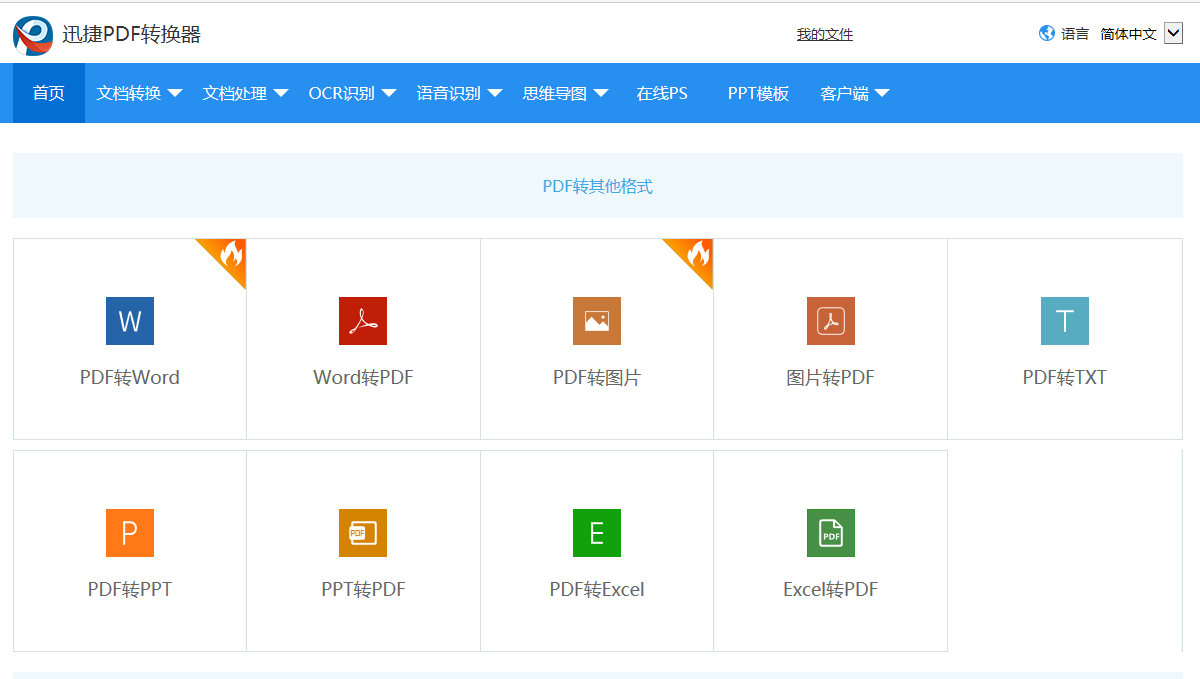２：在网站首页导航栏的位置找到OCR识别，点击OCR识别就会看到手写文字识别。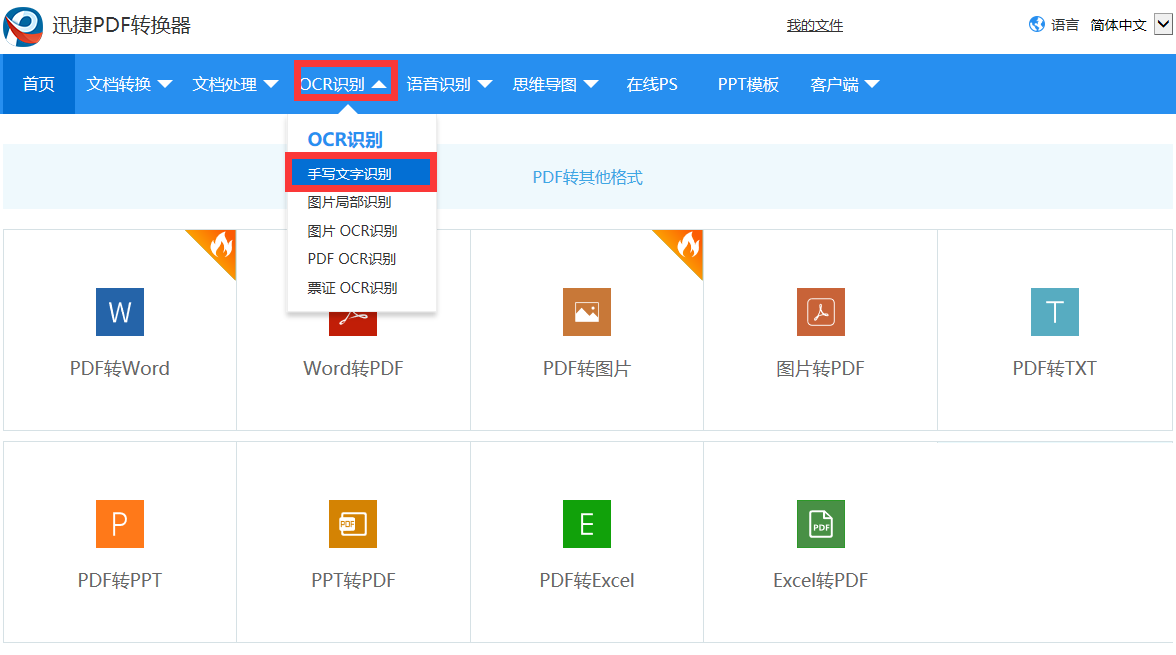３：点击手写文字识别进入网站的转换页面，进入转换页面，点击选择文件，选择你需要进行手写文字识别的文件，也可以直接将文件夹里面的文件拖进转换页面。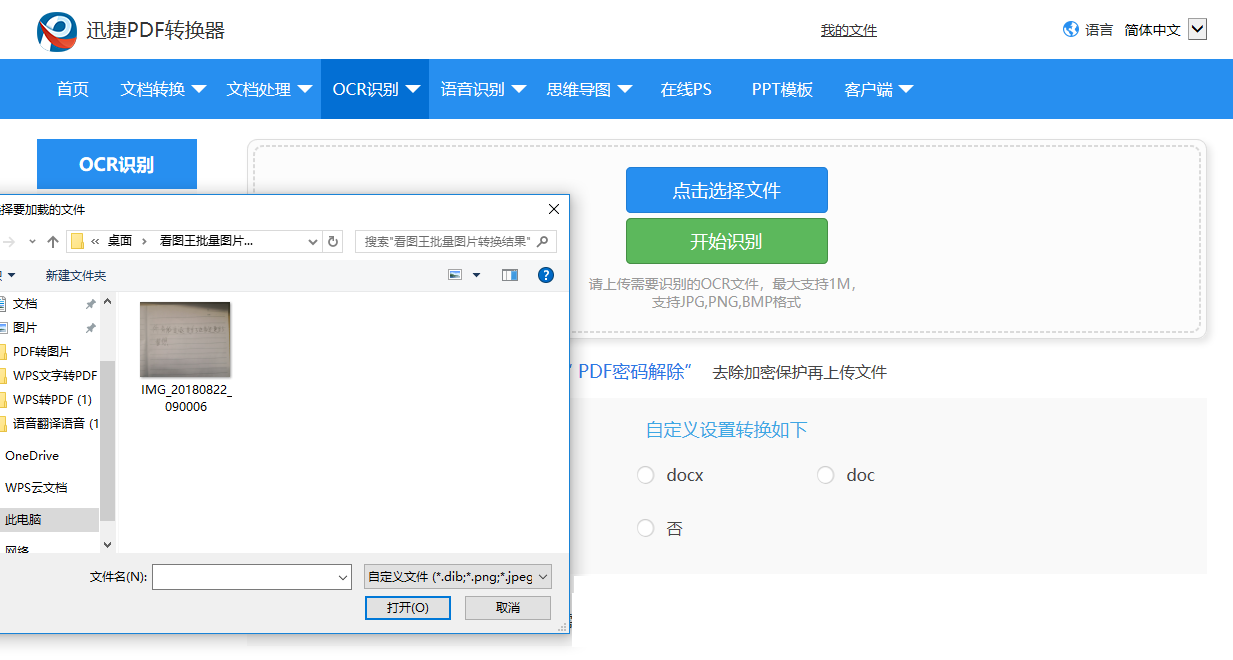４：点击开始识别，你需要进行手写文字识别的文件就会在识别的过程中，请耐心等待。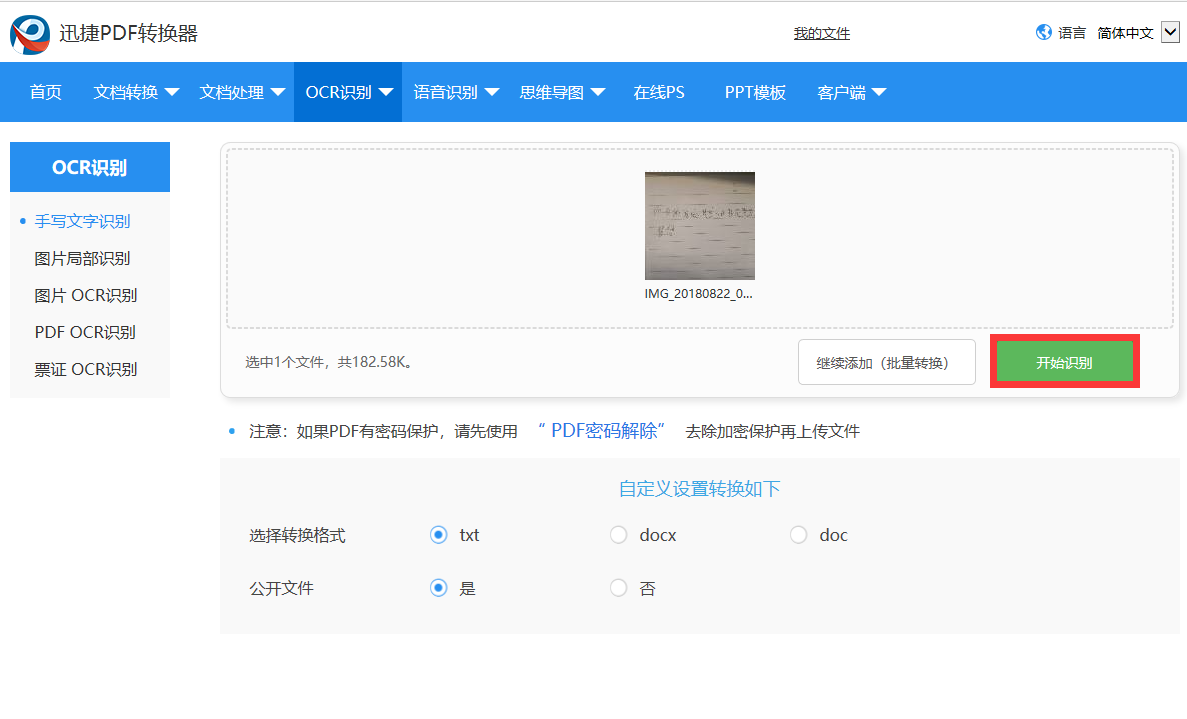５：识别完成的文件可以在导航栏上面的我的文件里保存查看，也可以直接下载到指定的文件夹中。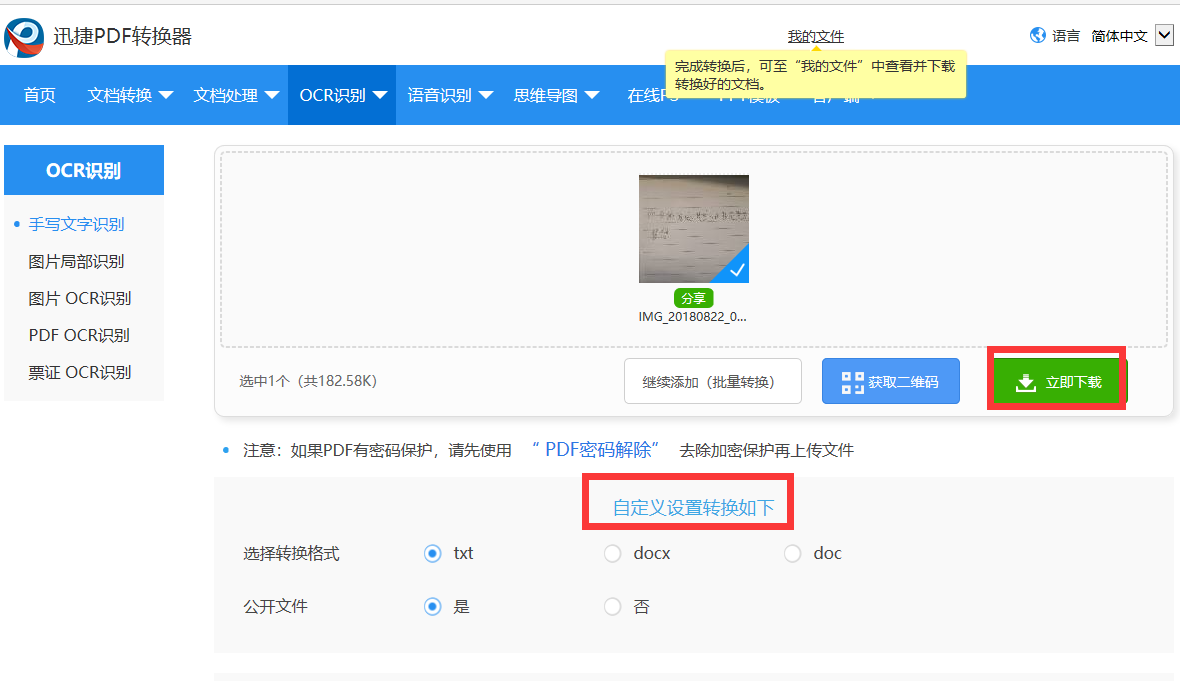分享给大家的手写文字识别的软件和操作方法就完成了，你学会了吗？
迅捷PDF在线转换器http://app.xunjiepdf.com/

转载于:https://my.oschina.net/u/3913465/blog/1931996
展开全文• ## Tensorflow 实现 MNIST 手写数字识别

万次阅读 热门讨论 2017-04-09 16:05:52
本节笔记作为 Tensorflow 的 Hello World，用 MNIST 手写数字识别来探索 Tensorflow。笔记的内容来自 Tensorflow 中文社区和黄文坚的《Tensorflow 实战》，只作为自己复习总结。
本节笔记作为 Tensorflow 的 Hello World，用 MNIST 手写数字识别来探索 Tensorflow。笔记的内容来自 Tensorflow 中文社区和黄文坚的《Tensorflow 实战》，只作为自己复习总结。
环境：
Windows 10
Anaconda 4.3.0
Spyder
本节笔记主要采用 Softmax Regression 算法，构建一个没有隐层的神经网络来实现 MNIST 手写数字识别。
1. MNIST 数据集加载
MNIST 数据集可以从MNIST官网下载。也可以通过 Tensorflow 提供的 input_data.py进行载入。
由于上述方法下载数据集比较慢，我已经把下载好的数据集上传到CSDN资源中，可以直接下载。
将下载好的数据集放到目录C:/Users/Administrator/.spyder-py3/MNIST_data/下。目录可以根据自己的喜好变换，只是代码中随之改变即可。
通过运行Tensorflow 提供的代码加载数据集：
from tensorflow.examples.tutorials.mnist import input_data

# 获取数据

MNIST数据集包含55000样本的训练集，5000样本的验证集，10000样本的测试集。 input_data.py 已经将下载好的数据集解压、重构图片和标签数据来组成新的数据集对象。
图像是28像素x28像素大小的灰度图片。空白部分全部为0，有笔迹的地方根据颜色深浅有0~1的取值，因此，每个样本有28x28=784维的特征，相当于展开为1维。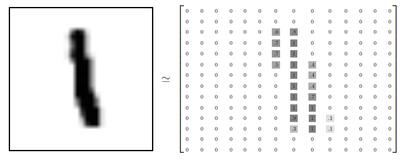所以，训练集的特征是一个 55000x784 的 Tensor，第一纬度是图片编号，第二维度是图像像素点编号。而训练集的 Label（图片代表的是0~9中哪个数）是一个 55000x10 的 Tensor，10是10个种类的意思，进行 one-hot 编码 即只有一个值为1，其余为0，如数字0，对于 label 为[1,0,0,0,0,0,0,0,0,0]。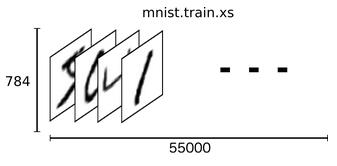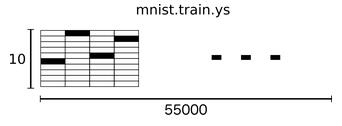2. Softmax Regression 算法
数字都是0~9之间的，一共有10个类别，当对图片进行预测时，Softmax Regression 会对每一种类别估算一个概率，并将概率最大的那个数字作为结果输出。
Softmax Regression 将可以判定为某类的特征相加，然后将这些特征转化为判定是这一个类的概率。我们对图片的所以像素求一个加权和。如某个像素的灰度值大代表很有可能是数字n，这个像素权重就很大，反之，这个权重很有可能为负值。
特征公式：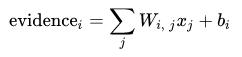bib_i 为偏置值，就是这个数据本身的一些倾向。
然后用 softmax 函数把这些特征转换成概率 yy :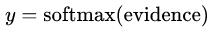对所有特征计算 softmax，并进行标准化（所有类别输出的概率值和为1）：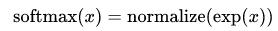判定为第 i 类的概率为：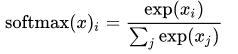Softmax Regression 流程如下：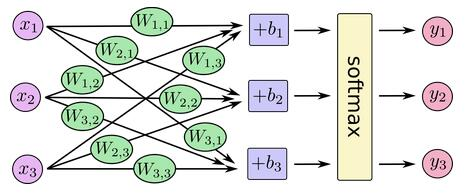转换为矩阵乘法：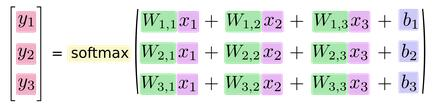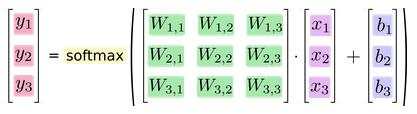写成公式如下：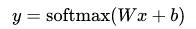3.实现模型
import tensorflow as tf
sess = tf.InteractiveSession()
x = tf.placeholder(tf.float32, [None, 784])
W = tf.Variable(tf.zeros([784,10]))
b = tf.Variable(tf.zeros())
y = tf.nn.softmax(tf.matmul(x,W) + b)

首先载入 Tensorflow 库，并创建一个新的 InteractiveSession ，之后的运算默认在这个 session 中。
placeholder：输入数据的地方，None 代表不限条数的输入，每条是784维的向量
Variable：存储模型参数，持久化的
4.训练模型
我们定义一个 loss 函数来描述模型对问题的分类精度。 Loss  越小，模型越精确。这里采用交叉熵：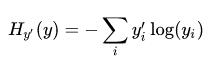其中，y 是我们预测的概率分布, y’ 是实际的分布。
y_ = tf.placeholder(tf.float32, [None,10])
cross_entropy = tf.reduce_mean(-tf.reduce_sum(y_ * tf.log(y),reduction_indices=))

定义一个 placeholder 用于输入正确值，并计算交叉熵。
接着采用随机梯度下降法，步长为0.5进行训练。
train_step = tf.train.GradientDescentOptimizer(0.5).minimize(cross_entropy)

训练模型，让模型循环训练1000次，每次随机从训练集去100条样本，以提高收敛速度。
for i in range(1000):
batch_xs, batch_ys = mnist.train.next_batch(100)
train_step.run({x: batch_xs, y_: batch_ys})

5.评估模型
我们通过判断实际值和预测值是否相同来评估模型，并计算准确率，准确率越高，分类越精确。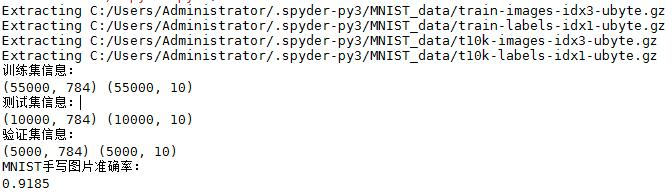6.总结
实现的整个流程：
定义算法公式，也就是神经网络前向传播时的计算。
定义 loss ，选定优化器，并指定优化器优化 loss。
迭代地对数据进行训练。
在测试集或验证集上对准确率进行评测。
7.全部代码
import tensorflow as tf

from tensorflow.examples.tutorials.mnist import input_data

# 获取数据

print('训练集信息：')
print(mnist.train.images.shape,mnist.train.labels.shape)
print('测试集信息：')
print(mnist.test.images.shape,mnist.test.labels.shape)
print('验证集信息：')
print(mnist.validation.images.shape,mnist.validation.labels.shape)

# 构建图
sess = tf.InteractiveSession()
x = tf.placeholder(tf.float32, [None, 784])
W = tf.Variable(tf.zeros([784,10]))
b = tf.Variable(tf.zeros())

y = tf.nn.softmax(tf.matmul(x,W) + b)

y_ = tf.placeholder(tf.float32, [None,10])
cross_entropy = tf.reduce_mean(-tf.reduce_sum(y_ * tf.log(y),reduction_indices=))

# 进行训练
tf.global_variables_initializer().run()

for i in range(1000):
batch_xs, batch_ys = mnist.train.next_batch(100)
train_step.run({x: batch_xs, y_: batch_ys})

# 模型评估
correct_prediction = tf.equal(tf.argmax(y,1), tf.argmax(y_,1))
accuracy = tf.reduce_mean(tf.cast(correct_prediction, tf.float32))

print('MNIST手写图片准确率：')
print(accuracy.eval({x: mnist.test.images, y_: mnist.test.labels}))



展开全文Tensorflow
• 利用matlab制作的手写数字识别系统、代码可读性强，结构清晰、GUI运行界面、在Matlab R2014b下编辑完成，有手写版，也可导入图片识别
• TensorFlow实战教程，该课程主要分享两个TensorFlow卷积神经网络实战的案例，第一个是手写数字识别案例，第二个是人脸识别案例。深度学习 卷积神经网络
• 为了使机器更智能，开发人员正在研究机器学习和深度学习技术。人类通过反复练习和重复执行任务来学习执行任务，从而记住了如何执行任务。...手写数字识别是计算机识别人类手写数字的能力。对于机器而言，这是一项...
• 1联机手写数字识别设计一、设计论述模式识别是六十年代初迅速发展起来的一门学科。由于它研究的是如何用机器来实现人(及某些动物)对事物的学习、识别和判断能力，因而受到了很多科技领域研究人员的注意，成为人工...
• 看了上一篇内容之后，相信对K近邻算法有了一个清晰的认识，今天的内容——手写数字识别是对上一篇内容的延续，这里也是为了自己能更熟练的掌握k-NN算法。我们有大约2000个训练样本和1000个左右测试样本，训练样本...
• ## MNIST 手写数字识别（一）

万次阅读 多人点赞 2018-11-07 21:43:33
MNIST 手写数字识别模型建立与优化 本篇的主要内容有： TensorFlow 处理MNIST数据集的基本操作 建立一个基础的识别模型 介绍 SoftmaxSoftmaxSoftmax回归以及交叉熵等 MNIST是一个很有名的手写数字识别数据集（基本...MNIST
• ## MATLAB手写数字识别

千次阅读 2020-01-02 16:58:38
MATLAB手写字体识别 使用svm进行手写字体识别 测试图片 处理图片，拿到数字区域并分割保存 clc,clear,close all; f=imread('num.jpg'); bw=~im2bw(f); se=strel('line',200,0); bw_dilate=imdilate(bw,...matlab python...